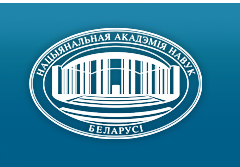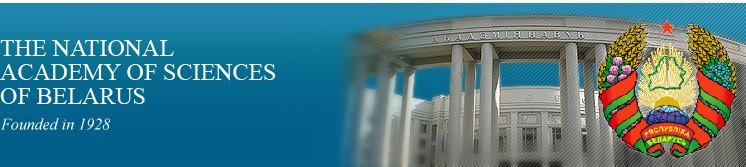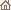Home News About Academy Academy Activities Academy Membership Organizations Publications Conferences Contacts Site Map

# Proc. of the Natl. Academy of Sciences of Belarus, Ser. Phys.-Math. Sci., 2003, No.1/ Publications / Scientific Journals

Proc. of the Natl. Academy of Sciences of Belarus, Ser. Phys.-Math. Sci., 2003, No.1Proceedings of the National Academy of Sciences of Belarus (Vestsi Natsiyanalnai Akademii Navuk Belarusi) SERIES OF PHYSICAL-MATHEMATICAL SCIENCESPublished by The Institute of Mathematics of NASB, Minsk, Republic of Belarus

### Number 1, 2003## CONTENTS

### MATHEMATICS

Alekhno E. A., Zabreyko P. P.
Quasi-positive elements and non-decomposible operators in ideal spaces. II. pp. 5--10

Summary: The class of non-decomposable linear integral operators in ideal spaces is studied. The positiveness of spectral radius of these operators is proved, their basic spectral properties are described and the theorem about general form of a kernel of the imprimitive non-decomposable operator is obtained.

Kharin Yu. S., Pashkevich M. A.
Statistical Estimation of the parameters of the beta-binomial distribution in the case of distortions of binary observations. pp. 11--17

Summary: The robustness problems of estimation of parameters for the beta binomial distribution (BBD) in the case of distortions of binary observations is considered. It is proved that the moment estimator and the maximum likelihood estimator are inconsistent in the case of binary data distortions. A new consistent moment estimator of the BBD parameters in the case of known distortion levels is developed. An iterative algorithm for joint estimation of BBD parameters and distortion levels is also proposed. Computer simulation results are also given,

Malyutin V.B.
Evaluation of functional integrals with respect to space offunctions with finite number of values. pp. 18--21

Summary: The class of functional integrals with respect to space of functions taking values ±l is evaluated,

Beresnevich V. V.
On the construction of regular systems of points with real, complex and p-adic algebraic coordinates. pp. 22--27

Summary: The method of constructing regular systems of algebraic points in the space of R x C x Qp is proposed.

Shabalina I. P.
On the lattice of t-closed n-multiply w-local formations of finite groups. pp. 28--30

Summary: It is proved that the lattice of t-closed n-multiply w-local formations is modular for all natural n.

Kuzmenkova I. A.
Radical ideal subgroup functors and Fitting classes. pp. 31--33

Summary: The concept of radical t-ideal subgroup functor is introduced. Isomorphism of both lattices of all radical t-ideal subgroup functors and of all Fitting classes is established.

Yudin M. D.
About indispensable conditions of convergence of distributions of the sums of dependent random vectors. pp. 34--37

Summary: The indispensable conditions of convergence of distributions of the sums of dependent random vectors to is boundless to divided distributions are considered.

Primicheva Z. N.
Coefficient conditions of dichotomy for triangular systems. pp. 38--44

Summary: The sets of Lp-dichotomic, p w 1, or exponentially dichotomic linear systems with supplementary projections E and O are considered. Coefficient conditions of the dichotomy for linear triangular systems are established.

Krasovskaya T. G.
The structure of the set of lower Perron exponents of infinitely differentiable linear system. pp. 45--49

Summary: It is proved that the set S Î R is the set of lower Perron exponents of the linear differential system x. = A(t)x, x Î R<>n, t ³ 0, with infinitely differentiable bounded coefficients and their bounded derivatives of any order, if and only if the S is a bounded Suslin set and sup S Î S.

Tretyakova N. N.
Limit correlations between some integral transforms in Euclidean space. pp. 50--55

Summary: Two new types of integral transforms which are generalize Fourier, Fourier -- Bessel, Hankel transforms are introduced. The conditions of their convergence are established. The limit relations between transforms introduced are obtained. This result has many interesting consequences.

Audoshka I. V.
Asymptotic integration of the dynamical equations of shallow shells subjected to external forces. pp. 56--61

Summary: By using the complex WKB-method, the formal asymptotic solution of the Cauchy problem for a set of partial differential equations describing motion of thin shell of arbitrary form is found. It is supposed that initial displacements and velocities of surface points are localized near some point on a shell surface.

### PHYSICS

Bogush A. A., Kisel V. V., Tokarevskaya N.G., Red'kov V. M.
On equations for the spin-2 particle in external electromagnetic and gravitational fields. pp. 62--67

Summary: 30-component, of the first order, equation by Fedorov -- Krylov -- Regge for the spin 2 particle, equivalent to the second order Pauli -- Firz one, is generalized to presence of an external electromagnetic field as well as a curved background space-time geometry. The essential property of the generally covariant wave equation obtained is that here from the very beginning, in accordance with requirement of the Pauli -- Firz approach, a set of additional relations on 30-component wave function for eliminating complementary spin 0 and spin 1 fields is present at the starting equation.

Pilipovich V. A., Esman A.K., Goncharenko I. A., Kuleshov V.K., Sergienya S.A.
Parallel optical adder based on fibre logic elements with Bragg reflectors. pp. 68--71

Summary: Gaussian's functions are used to consider properties of optical spatial-temporal solitons propagating in inhomogeneous elliptical waveguide with Kerr local nonlinearity. It is shown that the same as in the spherically symmetrical case both transverse dimensions and temporal duration of the light bullet oscillates along with the pulse propagating in the waveguide.

Blinkouski A. A., Gorbatsievich A.K.
Construction of exact cosmological solutions in the fivedimensional Projective Unified Field Theory. pp. 72--75

Summary: In the framework of the five-dimensional Projective Unified Field Theory in which gravitation is described by both space-time curvature and some hypothetical scalar field, we propose a method for construction of exact solutions for homogeneous and isotropic cosmological models when the time dependence of mass density is given. General consequences of this approach are investigated and new exact solutions are produced.

Komarov F.F., Vlasukova L.A., Mironov A. M., Komarov A. A., Yuvchenko V. N.
Redistribution of indium implanted in silicon under the influence of thermal treatment and superhigh-energy ion irradiation. pp. 76--80

Summary: The layer of supersaturated solid solution with uniform concentration of introduced In atoms up to ~ 5 at. % and thickness up to ~ 150 nm had been created in Si by polyenergetic implantation. It has been observed close correlation of experimental In distribution profile along the depth of implanted crystal and computer calculations. The implanted samples had been annealing at 550°C during 35 minutes and then had been irradiated by 710 MeV -- Bi with fluences 1 x 1011 and 1 x 1012 cm-2. The introduced atoms redistribution under the post-implanted annealing and followed irradiation by swift Bi ions had been investigated by means of Rutherford backscattering/channeling. The significant redistribution of indium concentration at subsurface and peripheral regions of profile has been observed both after annealing and after additional irradiation by super-high energy Bi ions. The effect is increased with the growth of Bi ions dose.

Tsionenko D. A.
The Dirac--Kahler equation in a non-Euclidean space-time. pp. 81--85

Summary: The matrix formulation of the Dirac--Kahler equation in a curved space-time is constructed. The Lagrange formulation is given. The conservation laws and symmetries of the theory in a non-Euclidean space-time are investigated.

Shipilo V. B., Dutov A.G., Shipilo N.V., Comar V. A., Kurilovich N. Ph., Azarco I. I., Shempel N. A.
Crystallization of diamonds in a melt of metal at use of graphite irradiated with gamma-quantums. pp. 86--90

Summary: The influence of an irradiation a gamma-quantums of reactionary mix material for synthesis of diamonds in a system Mn -- Ni -- C -- Cu on a mass fraction and speed of transformation of graphite in diamond is investigated at P = 5.5 GPa, T = 1620 K and t = 150 s, grit size and admixture makeup of diamond. It is shown that an irradiation of mix material the gamma-quantums diminishes an exit and speed of transformation of graphite in diamond and also contents in diamonds of manganese and nickel. Thus the density of paramagnetic imperfections is essentially augmented. The obtained experimental data are considered from a point of view of the colloidal theory of synthesis of diamonds and contact to features of shaping of structure of carbon complexes in a melt of metal.

Solovyh T. K., Truhanov S. V., Troyanchuk I. O., Kasper N. V., Shimchak G., Berner K.
Magnetic phase transformations in anion deficit manganite La0.7Ca0.3MnO3-g, with structure of perovskite. pp. 91--96

Summary: The crystal structure, magnetic and electrical properties of the La0.70Ca0.30MnO3-g (0 £ g £ 0.20) oxygen-deficient manganites have been studied. It is found that the compounds La0.70Ca0.30MnO3-g show a long-range ferromagnetic order up to g = 0.06 and a cluster spin glass behavior at 0.06 < g < 0.20. The strongly reduced samples exhibit a large magnetoresistance below the temperature, at which the spontaneous magnetization develops. The magnetic phase diagram of La0.70Ca0.30MnO3-g system has been constructed. We argue, that the oxygen vacancies are disordered in the La0.70Ca0.30MnO3-g system in the studied region of oxygen vacancies concentration (0 £ g < 0.20).

Kazakevich L.A., Lugakov P.F.
Recombination of charge carriers on radiation-induced defects in silicon doped by transition metals impurities. pp. 97--100

Summary: It has been studied the peculiarities of recombination of nonequilibrium charge carriers on radiation-induced defects in received according to Chohralsky method p-silicon (p » 3 - 20 Ohm • cm), doped by one of the impurities of transition metals of the IV-th group of Mendeleev table (titanium, circonium, hafnium). Experimental results are obtained out of the analysis of temperature and injection dependence of the life time of charge carriers. The results are explained taking into consideration the influences of elastic stress fields created by the aggregates of transition metals atoms on space distribution over the crystal of oxygen and carbon background impurities as well as on the migration of movable radiation-induced defects during irradiation.

### INFORMATICS

Bakhanovich S.V., Likhoded N. A.
Mapping algorithms onto fixed dimension and fixed size parallel architecture. pp. 101--108

Summary: The problem of space-time mapping of algorithms with uniform dependencies onto the given dimension parallel architecture with given number of processors is researched. The investigations is developed in the framework of locally parallel globally sequential strategy. This paper extends previous investigations: the dimensions n and p of algorithm and parallel architecture, respectively, are arbitrary, but p < n.

Evdokimovich V. E.
The closed queueing networks with dynamic routing and dynamic probability rounds of nodes by the customers. pp. 109--113

Summary: The closed exponential networks with routing matrix depending on their state are considered. The customers arriving in a node, irrespective of other customers with probability depending on a network state, join queue, or with additional probability instantly round a node. After detour of a node customers move according to stochastic matrix depending on a network state and, generally speaking, distinct from routing matrix. The sufficient condition of an ergodicity is installed with certain restrictions on model parameters and the final stationary distribution is founded.

### SHORT NOTES

Bogush A. A.
On two kinds of the vector parametrization of the three-dimensional rotation group. pp. 114--117

Summary: The basic relations obtained in the framework of two different vector parametrizations of the rotation group (1958, 1977, Fedorov; 2001, Engo) are compared.

### ABSTRACTS OF DEPOSITED PAPERS

Lukshin E.V.
About the types of (3n - 3) nonintegral vertices of polyhedral of three indexed, axial assignment problem. p. 118

### SCIENTISTS OF BELARUS

Andrey Markovich Goncharenko (On the occasion of the 70th birthday). pp. 119--120
Click
here to read this article in Russian (Windows 1251)

Anton Nikiforovich Sevchenko (On the occasion of the 100th birthday). pp. 121--123
Click here to read this article in Russian (Windows 1251)/ Publications / Scientific Journals / Back to Top of Page

Designed and maintained by Dr. Nikolai N. Kostyukovich. Last updated: May 3, 2003
Created with assistance of Dr. Ignatii I. Korsak
Copyright © 2003 The National Academy of Sciences of Belarus
Copyright © 2003 The Institute of Mathematics of NASB